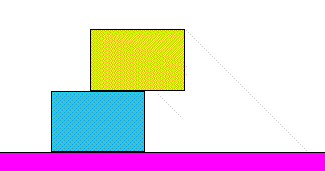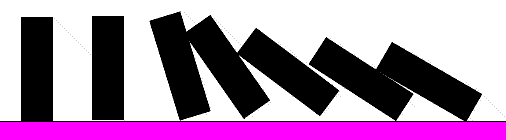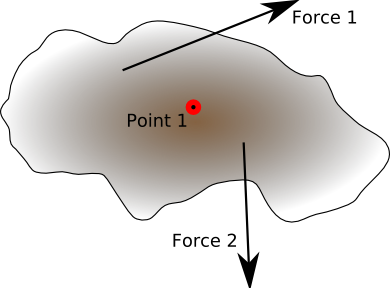# 3D Physics - Forces

This page covers static forces, if the objects start moving then we need to use differential equations.

In most 3d modeling programs, shapes can be moved around and will be able to pass through each other. However in a physics modeling program we need a way to prevent solid objects from occupying the same space. We also need to know whether objects are in equilibrium, or whether they are going to start accelerating in some direction. To do this, we need to model forces. Is there any way that this can be done by the program itself from its database of the properties of objects in its world, without being explicitly told about where the forces are acting?

This seems like a difficult problem and this page tries to work out some of the general rules. If this approach is not possible then it may be simpler to model the system by explicitly defining a framework of solid objects and joints as on the next page.

### Forces

Forces acting on the 'centre of mass' c-of-m can be added using vector addition. Torque's (Force times distance) tending to cause rotation can also be added.

### Gravity

This acts on the c-of-m in the -y direction. If an object is resting on the earth (for this purpose assume the earth is an object with infinite mass) and its c-of-m is above its base, then there is not much point in working out the forces (unless there is enough sideways force to overcome friction or knock it over) and the object can be marked as 'at rest'.

This also applies to objects on top of objects on the ground.When a sideways force is applied to an object on the ground, then it may slide depending on the friction coefficients, if not the force may be strong enough to tip it up. When the c-of-m is outside the base then it will start to fall.## Rotational Forces

The following terms are associated with rotational forces:

### Torque

When two, or more, forces are acting on a solid body and the forces are not acting in line then there may be an overall turning effect. Where a force tries to move an object in a linear way a torque tries to turn the object. The torque is measured about a particular point on a solid object. The magnitude of the torque, about this given point, depends both on the magnitude and direction of the forces and there distance from the point.### Couple or Moment

This is a system of forces that tries to produce a rotation only. That is the forces are balanced so that there is no overall linear force.

A system of forces, acting on a solid object, can be resolved into a couple and a linear force. If the resultant of all forces and couples is not zero the object will move.We can resolve an arbitrary system of forces into a single couple and single force.

• To find the resultant force we take the vector sum of the forces without taking account the points at which the force acts.
• To find the resultant couple, about some convenient point (point 1) such as the centre of mass, we take the vector sum of the cross product of the force with its distance from the point (F x r).

If the system of forces are in balance, then the point that we choose to measure the couple around is just a matter of choosing the most convenient point for the calculations. This is because, if it is in balance around one point, it is in balance around all points. However, if the forces are not in balance, it is important and we will want to find the axis where the couple can be consided to act.### Parallel Axis Theorm

We can convert one system of forces into an equivalent system of forces.

### Screws

A system of forces can be represented by Screw Theory. This allows us to apply transforms to the combination of linear and rotational quantities is a simpler way.

Combined Linear and Rotational Quantity Represents Requires
wrenches force and torque two vectors
twists velocity four line coordinates

## Other Forces

Other forces may be modeled, such as springs, electromagnetic, etc. These are covered in further sections.

### Forces

```forces { field SFFloat mass 1 field
SFVec3f CentreOfGravity 0 0 0 field SFNode follow field SFFloat magnetN 1 field
SFFloat magnetS 1 field SFFloat coefficientOfFriction } ```

• If the mass != 0 then the object will accelerate toward the ground.
• If follow != 0 then the object will accelerate toward another object.
• If Magnet != 0 the the object will be attracted/repelled to other magnets

## Programming StaticsFrom the third law, we know that forces always come in pairs, so we need to hold forces in a separate structure which is associated with two objects. This ensures that the magnitude of the force only needs to be stored once. Strictly gravity should also be associated with two objects, in the same way as the contact forces are, however in the case of gravity the other object is usually the earth, so it is probably more efficient to treat this as a special case.

For a multiple object simulation such as this then, we might start by looking for an object where the external forces can be determined, in the above example, none of the objects are independent. So we may have to setup a set of equations to determine the inertia force on each object in terms of the forces on the other objects.

Where I can, I have put links to Amazon for books that are relevant to the subject, click on the appropriate country flag to get more details of the book or to buy it from them.

Commercial Software Shop

Where I can, I have put links to Amazon for commercial software, not directly related to the software project, but related to the subject being discussed, click on the appropriate country flag to get more details of the software or to buy it from them.Dark Basic Professional Edition - It is better to get this professional editionThis is a version of basic designed for building games, for example to rotate a cube you might do the following: make object cube 1,100 for x=1 to 360 rotate object 1,x,x,0 next x

This site may have errors. Don't use for critical systems.